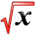# Differential Equations ExamplesThis article is part of the MathHelp Tutoring Wiki

## Initial Value Problem

Example 1 :
Question:

Solve the following initial value problem.
$y'=3t^{2}-4$, y(0) = 5
Given that the general solution is $f(t)=t^{3}-4t+C$Solution:
Since we know y(0) = 5.
That means t = 0.
Therefore $f(0)=0^{3}-4(0)+C=5$$0-0+C=5$$C=5$The desired solution is $f(t)=t^{3}-4t+5.$Example 2 :
Question:

Solve the following initial value problem.
$y'=2y$, y(0) = 3
Given that the general solution is $y=Ce^{2t}$Solution:
Since we know y(0) = 3.
That means t = 0.
Therefore $y(0)=Ce^{2(0)}=3$$C(1)=3$$C=3$The desired solution is $y=3e^{2t}.$## First-Order Linear Differential Equations

To solve first-order differential equations, you'll need to know the following facts:
• A first-order linear differential equation in standard form is y' + a(t)y = b(t).
• Find an integrating factor by integrating e^(∫a(t)dt) which will become the integrating factor in the form $e^{A(t)}$.
• After find the integrating factor, multiply the first-order linear differential equation by the integrating factor.
• Then be able to recognize how to combine terms in y' and y into a single term after multiplying the differential equation by the integrating factor, for example:
1. $e^{t}y'+e^{t}y={\frac {d}{dt}}(e^{t}y)$2. $t^{2}y'+2ty={\frac {d}{dt}}(t^{2}y)$• After combining the terms into a single term, integrate both sides to solve for y.
• By solving y, you can find the general solution for y.

Example 1 :
Question:

Solve $t^{2}y'+ty$.
Solution:
Divide both sides by $t^{2}$to ensure the differential equation is in its standard form (y' + a(t)y = b(t)).
So the differential equation turns into $y'+{\frac {1}{t}}y={\frac {2}{t^{2}}}$Find the integrating factor by integrating e^(∫a(t)dt) = e^(∫${\frac {1}{t}}dt)=e^{ln(t)}$Then the integrating factor is $e^{A(t)}=e^{ln(t)}=t$.
Multiply the differential equation by the integrating factor t.
$(t)y'+(t){\frac {1}{t}}y=(t){\frac {2}{t^{2}}}=ty'+y={\frac {2}{t}}$Recognize that the left hand side has the terms y' and y and you can combine them into a single term.
After combining it into a single term, it is ${\frac {d}{dt}}(ty)={\frac {2}{t}}$.
You'll probably notice that you can combine the terms by recognizing that the single term is y times integrating factor = $e^{A(t)}$and in this example, it is yt.
Now integrate both sides and you'll get ty = ∫${\frac {2}{t}}dt=2lnt+C$.
Now isolate y and get $y={\frac {2lnt+C}{t}}$.
The general solution is $y={\frac {2lnt+C}{t}}$.

Example 2 :
Question:

Solve $y'+y=1$.
Solution:
We know that a(t) = 1 and b(t) = 1.
Integrating factor = e^(∫1dt) = $e^{t}$.
$e^{t}y'+e^{t}y=e^{t}$${\frac {d}{dt}}(e^{t}y)=e^{t}$$e^{t}y=$$e^{t}dt+C$$e^{t}y=e^{t}+C$$y={\frac {e^{t}+C}{e^{t}}}$$y=1+{\frac {C}{e^{t}}}$$y=1+e^{-t}C$The general solution is $y=1+e^{-t}C$or $y=1+{\frac {C}{e^{t}}}$.